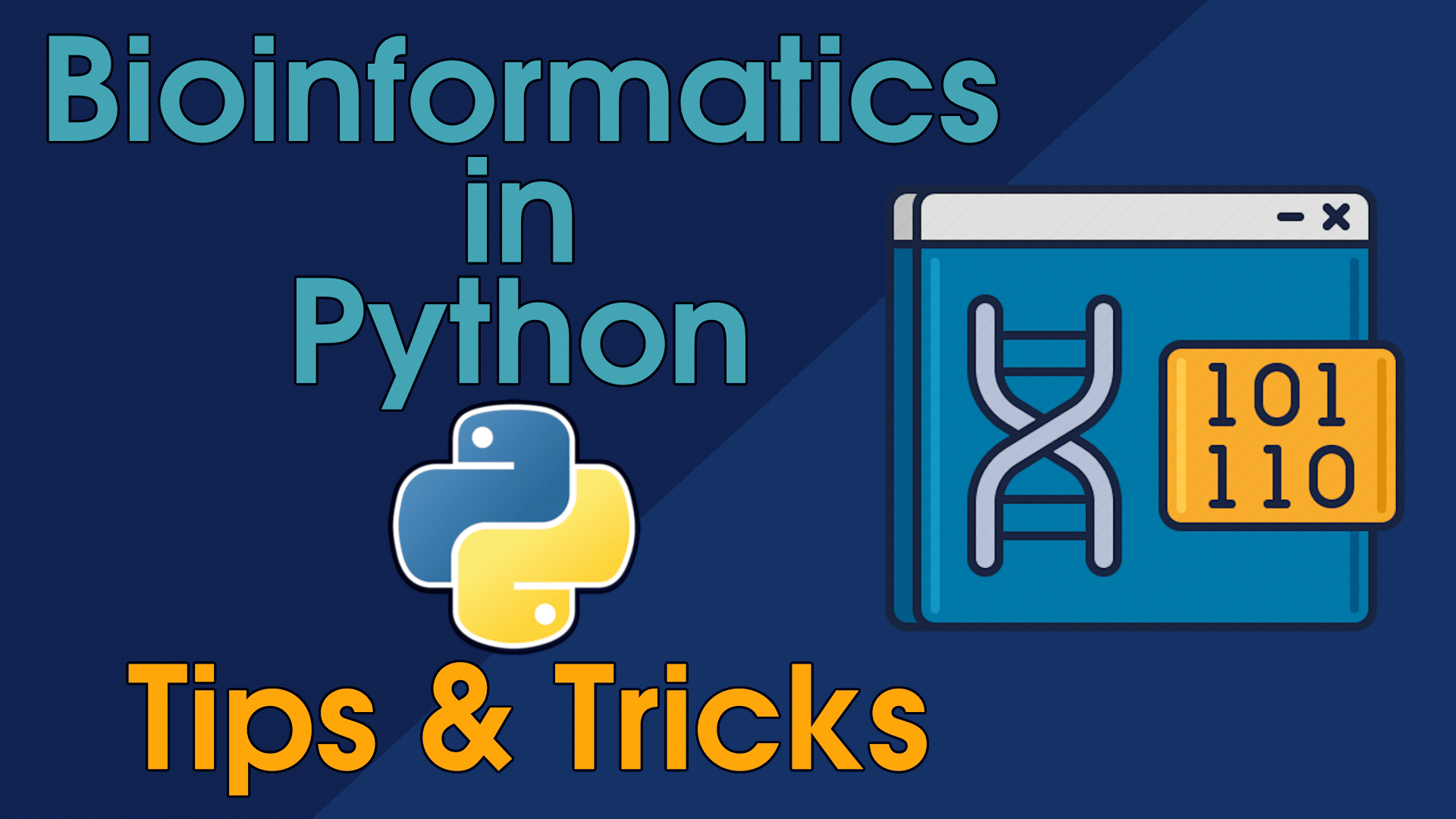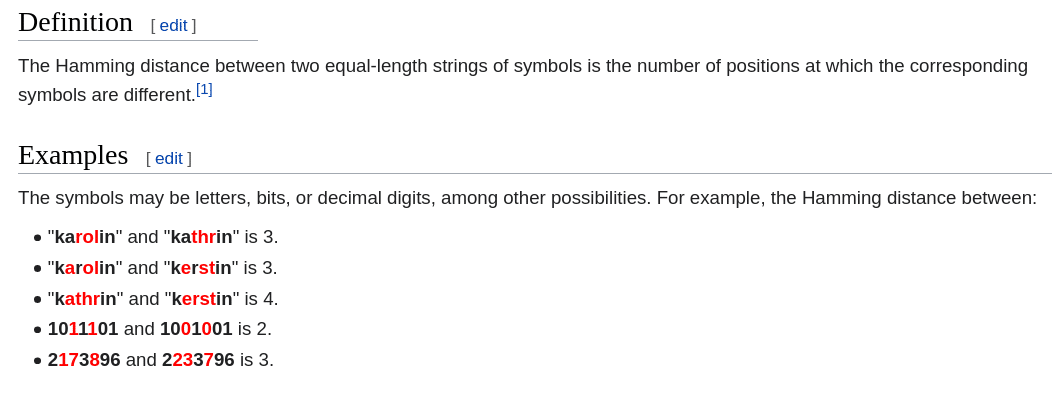# Tips & Tricks: Hamming DistanceLet’s take a look at how we can program Hamming Distance algorithm in three different ways.

We can use Hamming Distance to measure/count nucleotide differences in DNA/RNA. This is very useful when we are searching for a particular pattern in a genome with up to n mutations. If we found a pattern in a genome, that is responsible for some biological function, we can try searching for the same pattern in other related genomes. There is one problem though. Even if the same pattern exists and is present in the other genome, there is a huge probability it will have a mutation. It is also called SNP (Single-nucleotide polymorphism). Even though this part of a genome (one with a mutation) is a correct one, we will not find it if we search for it as a sub-string in a string. We need to search for a sub-string with up to n mutations in a string. We will discuss pattern, also called a motif, search algorithm in another article. Hamming Distance will help us with that. So let’s implement it.

Here is a Wikipedia image with a brief explanation and examples:https://en.wikipedia.org/wiki/Hamming_distance

Example: Hamming Distance between ATCGATCG and ATCCATGG is 2.

Let’s start by implementing two DNA strings with 4 mismatches:

```dna_str_1 = "TTCGATCCATTG"
dna_str_2 = "ATCAATCGATCG"```

`TTCGATCCATTGATCAATCGATCG`
First algorithm we will implement will be a simple, naive `for loop` solution:

```# Loop approach
def h_d_loop(str_1, str_2):
h_distance = 0
for position in range(len(str_1)):
if str_1[position] != str_2[position]:
h_distance += 1
return h_distance```

This approach is very simple, self-explanatory and beginner-friendly. We just loop through a length of one of the strings and compare characters of each string, one-by-one. If characters don’t match, we add 1 to h_distance variable which our function returns in the end.

Please note that this algorithm is only applicable in a case, when strings are of equal length. If they are not, we need to use a different algorithm. We will discuss that algorithm in a future article.

Let’s print out the result of this algorithm after we provide our two test DNA strings to it:

```print("Loop Hamming Distance: ", end='')
print(h_d_loop(dna_str_1, dna_str_2))```

The output should be: Loop Hamming Distance: 4

`end=''` part is one of the parameters print() method has, and by setting it to ‘ ‘ we are telling it “don’t go to a new line, after you print the message”. Because of that we see the output 4 on the same line as the text, and not on a new line. If you are not sure what this does, try removing this parameter or changing `end=''` to `end=' * '`. Experiment!

Now let’s look at set() approach:

```# Set Approach
def h_d_set(str_1, str_2):
nucleotide_set_1 = set([(x, y) for x, y in enumerate(str_1)])
nucleotide_set_2 = set([(x, y) for x, y in enumerate(str_2)])

return len(nucleotide_set_1.difference(nucleotide_set_2))```

This version is ‘cleaner’ but a bit harder to read. Set is a very useful data structure. If you never used it, I suggest looking into it. In the code above we use a list comprehension to generate two sets with enumeration. The best way to see what is being generated is to debug the code, or use a simple print() method. Let’s add a `for loop` that will print out both sets for us.

Add this code on the line 5 in the code above:

```for nuc in range(len(str_1)):
print(sorted(nucleotide_set_1)[nuc],
sorted(nucleotide_set_2)[nuc])```

We are using the length of one of the strings, as they both are the same length anyway, and we print both sets side-by-side. We also need to use a `sorted()` method as one of the properties of a set is that it stores data in a random order. Here is what we will see:

```(0, 'T') (0, 'A')
(1, 'T') (1, 'T')
(2, 'C') (2, 'C')
(3, 'G') (3, 'A')
(4, 'A') (4, 'A')
(5, 'T') (5, 'T')
(6, 'C') (6, 'C')
(7, 'C') (7, 'G')
(8, 'A') (8, 'A')
(9, 'T') (9, 'T')
(10, 'T') (10, 'C')
(11, 'G') (11, 'G')```

We can see mismatches on lines 1, 4, 8 and 11.

Last line in our `set()` algorithm uses a `difference()` method that set has. It returns a new set, that contains only mismatches in both sets.

If we try printing out that new set:

`print(nucleotide_set_1.difference(nucleotide_set_2))`

We will see this output, which is of length 4:

`{(3, 'G'), (0, 'T'), (10, 'T'), (7, 'C')}`

Now we just return the length of that new `difference()` based set.

Let’s also add the output to our file for this algorithm:

```print("Set Hamming Distance: ", end='')
print(h_d_set(dna_str_1, dna_str_2))```

If we run our code now, we will see two outputs:

`Loop Hamming Distance: 4Set Hamming Distance: 4`

And the final version will use a zip() method. We will start with a 3 line function for simplicity’s sake, and combine it into one line of code later.

```# Zip Approach
def h_d_zip(str_1, str_2):
zipped_dna = zip(str_1, str_2)
mismatches = [(n1, n2) for n1, n2 in zipped_dna if n1 != n2]

return len(mismatches)```

Let’s look into `zipped_dna` variable by printing it’s contents:

```for x in zipped_dna:
print(x)```

We can now see that it creates a new list of sets for us:

```('T', 'A')
('T', 'T')
('C', 'C')
('G', 'A')
('A', 'A')
('T', 'T')
('C', 'C')
('C', 'G')
('A', 'A')
('T', 'T')
('T', 'C')
('G', 'G')```

Now we just use a list comprehension to go through each set in a list and check if characters in that set are not the same. If they are not, we add that set to our new list we called mismatches.

After we have a new mismatches list, we just return a length of that list, which will have 4 sets in it. Try printing out mismatches variable if you are not sure what is being stored in it.

Now we will combine all 3 lines into one return statement and add the third output:

```# Zip Approach
def h_d_zip(str_1, str_2):
return len([(n1, n2) for n1, n2 in zip(str_1, str_2) if n1 != n2])```
```print("Zip Hamming Distance: ", end='')
print(h_d_zip(dna_str_1, dna_str_2))```

Final output will look like this:

`Loop Hamming Distance: 4Set Hamming Distance: 4Zip Hamming Distance: 4`

#### Source code GitLab link:

https://gitlab.com/RebelCoder/bioinformatics_tips_tricks/-/blob/master/h_distance.py

#### Video version of this article:

Categories: Tips & Tricks

### 0 Comments

This site uses Akismet to reduce spam. Learn how your comment data is processed.